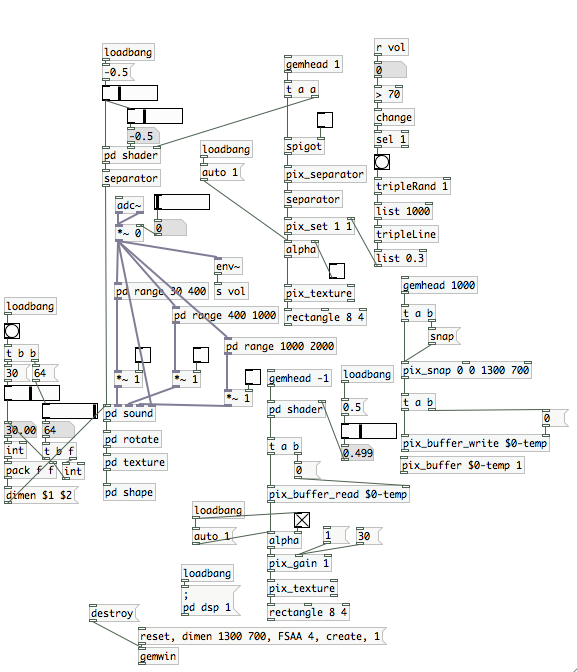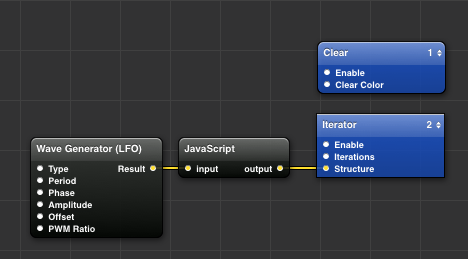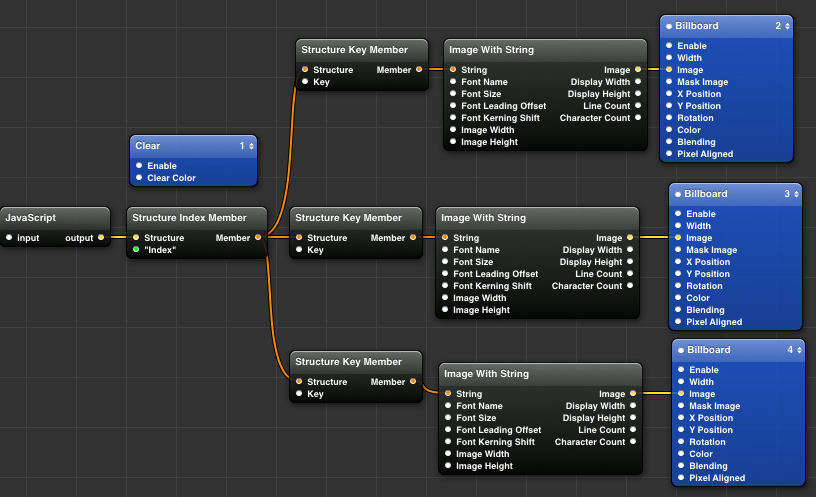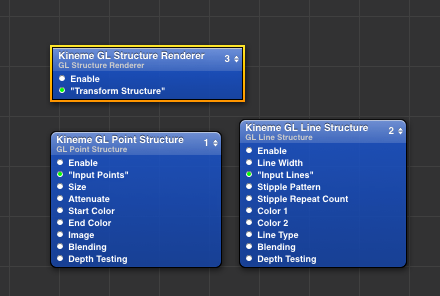## 2013年6月23日 星期日

### 「筆記」ＱＣ進階筆記 4-1

```var clicked=false;
var control={};
var clock=[];

function dis(x1,y1,x2,y2){
return Math.sqrt(Math.pow((x1-x2),2)+Math.pow((y1-y2),2));
}

function angle(x,y,x1,y1){
return Math.atan2(y1-y,x1-x);
}

function (__structure outlet,__structure outlet2) main (__number x,__number y,
__boolean click,__boolean reset,__number time)
{

for(var i=0;i<clock.length;i++){
clock[i].theta+=clock[i].r/10;
clock[i].x1=clock[i].x+Math.cos(clock[i].theta)*clock[i].r;
clock[i].y1=clock[i].y+Math.sin(clock[i].theta)*clock[i].r;
}

var result = new Object();
//if(click==true && clicked==false)
if(reset){
clock=[];
}

if(click && !clicked){
clicked=true;
control.posx=x;
control.posy=y;
control.enable=true;
}
if(click && clicked){
control.posx1=x;
control.posy1=y;
control.dis=dis(control.posx,control.posy,control.posx1,control.posy1);
control.angle=angle(control.posx,control.posy,control.posx1,control.posy1)
}
if(!click && clicked){
clicked=false;
control.enable=false;

clock.push({
x:control.posx,
y:control.posy,
x1:control.posx1,
y1:control.posy1,
r:control.dis,
theta:control.angle,
});
}
result.outlet=control;
result.outlet2=clock;
return result;
}
```

(draw line)

（iterator)

qc2-w4-1.qtz

## 2013年6月15日 星期六

### 「筆記」ＱＣ進階筆記3-2

```var lines=[];
function (__structure out) main (__number mousex,__number mousey,
__boolean click,__boolean reset,__boolean del,__boolean next)
{
var result = new Object();
if(click){
lines.push([mousex,mousey,0]);
}//按下滑鼠左鍵時，開始畫圖。

if(reset){
lines=[];
}//按下滑鼠右鍵時，清空畫布。

if(lines.length>100){
lines.shift();
}//線條長度大於100時，從尾巴遞減

if(del){
lines.pop();
}//按下鍵盤←鍵，刪除線條起始頭。

if(next){
lines.shift();
}//按下鍵盤→鍵，刪除線條尾巴。

result.out = lines;
return result;
}
```
qc2-w3-3.qtz

```var lines=[];

function (__structure out) main
(__number mousex,__number mousey,__boolean click,__boolean reset,__number timer)
{
var result = new Object();
for(var i=0;i<lines.length;i++){
lines[i] -= 0.01;
if(lines[i] < -2 ){
lines.splice(i,3);
}
}//當三角形的Ｚ軸位置小於-2時，刪除三角形。

if(click){
var size=Math.random()*0.2+0.01;
lines.push([mousex,mousey+size,0]);
lines.push([mousex+size,mousey,0]);
lines.push([mousex-size,mousey,0]);
}//點選滑鼠左鍵時，畫出一個三角形。

if(reset){
lines=[];
}//點選滑鼠右鍵時，清除所有的三角形。

result.out = lines;
return result;
}
```qc2-w3-4.qtz

### 「筆記」ＱＣ進階筆記3-1

```var line0=[
{X:0.5,Y:0,Z:0},
{X:0.1,Y:-0.4,Z:0},
{X:0.3,Y:0.2,Z:0},
{X:-0.2,Y:0.1,Z:0},
{X:-0.4,Y:0.3,Z:0},
];//陣列的宣告方式

var line1={
a1:{X:0.5,Y:0,Z:0},
a2:{X:0.1,Y:-0.4,Z:0},
a3:{X:0.3,Y:0.2,Z:0},
a4:{X:-0.2,Y:0.1,Z:0},
a5:{X:-0.4,Y:0.3,Z:0},
};//物件資料結構的宣告方式

function (__structure out) main (__number choice)
{
var result = new Object();
if(choice == 1){
result.out = line0;
}else{
result.out = line1;
}
return result;
}
```
qc2_w3-1.qtz

```function (__structure out) main (__number segments,__number size)
{
var result = new Object();
var lines=[];

for(var i=0;i<segments;i++){

var theta=(i/(segments-1))*Math.PI*2;
//example1
//lines[i]={X:Math.cos(theta)/size,Y:Math.sin(theta)/size,Z:0};
//物件的宣告方式

//example2
//lines[i]=[Math.cos(theta)/size,Math.sin(theta)/size,0];
//陣列的宣告方式

//example3
lines.push([Math.cos(theta)/size,Math.sin(theta)/size,0]);
}
//滑鼠的Ｘ軸方向，影響物件大小;y軸方向影響邊數。
result.out = lines;
return result;
}
```qc2-w3-2.qtz

## 2013年6月13日 星期四

### [作品]進擊的GLSL

Examole download

geometry的程式碼，參考來自Pd官方Help中的GEM>examples>glsl>geometry.pd
(subpath [pd shape1])

Examole downloadExamole download

## 2013年6月8日 星期六

### [教學]第一個GLSL的範例

Music:Giardini di Mirò- A new start

### vertex code

```uniform float vel;
uniform vec3 noise;
varying vec4 color;

void main()
{
vec3 pos = gl_Vertex.xyz + noise3( gl_Vertex.xyz * vel * noise) ;
gl_Position = gl_ModelViewProjectionMatrix * vec4(pos,1.0);
}

```

### fragment code

```uniform vec4 color;

void main()
{

gl_FragColor = color;
} ```Download example

## 2013年6月7日 星期五

### 「筆記」ＱＣ進階筆記 2-3

```var first=true;
var x1;
var y1;
var x2;
var y2;

var out_x;
var out_y;
var width;
var height;

var mySquare=[];

function (__number out_x,__number out_y,__number width,__number height,__structure myStructures)
main (__number x,__number y,__boolean click ,__boolean clear,__number time)
{
var result = new Object();
if(clear){ mySquare=[];}

if(click){
if(first){
x1=x;
y1=y;
first=false;
}
x2=x;
y2=y;

out_x=(x1+x2)/2;
out_y=(y1+y2)/2;
width=Math.abs(x1-x2);
height=Math.abs(y1-y2);

result.out_x=(x1+x2)/2;
result.out_y=(y1+y2)/2;
result.width=Math.abs(x1-x2);
result.height=Math.abs(y1-y2);

}else{
if(first==false){
mySquare.push({ax:out_x,ay:out_y,awidth:width,aheight:height});
}
first=true;
}

for(var i=0;i<mySquare.length;i=1+1){

if(mySquare[i].ay <2){
mySquare[i].ay += (i%15+5)/300;
}else{
mySquare[i].ay =-1
}
}
result.myStructures=mySquare;
return result;
}

```（主程式）

（NewSquare）

Download qc2_w2-5.qtz

### 「筆記」ＱＣ進階筆記 2-2

```function (__structure output) main (__number input)
{
var myStructure = [
{x:-0.1+input,y:-0.1,z:0,r:0.2},
{x:0.2+input,y:-0.5,r:0.15},
{x:-0.3+input,y:0.2,r:0.3},
{x:0.4+input,y:0.5,r:0.25},
] ;

var result = new Object();
result.output = myStructure;
return result;
}
```qc2_w2-3.qtz

```var myStructure = [
{X:-0.1,Y:-0.1,Z:0},
{X:0.2,Y:-0.5,Z:0},
{X:0.3,Y:0.2,Z:0},
{X:-0.4,Y:0.5,Z:0},
] ;

function (__structure output) main (__number input)
{
for(var i=0;i < myStructure.length ; i=i+1){
myStructure[i].Z += (i+1)/500;
myStructure[i].Z %= 1;
}
var result = new Object();
result.output = myStructure;
return result;
}
```

（主程式）(lighiting 物件內)

qc2_w2-4.qtz

### 「筆記」ＱＣ進階筆記 2-1

```var myStructure = [] ;
var state=true;
function (__number output,__number index) main (__number input)
{
if(state==true){
for(var i=0;i<10;i++){
myStructure[i]=Math.floor(100*(Math.random()));
}
}
state=false;
var result = new Object();
result.output = myStructure[Math.floor(input)];
result.index=Math.floor(input);
return result;
}
```Download qc2_w2-1.qtz

```var myStructure =
[
{x:0.1,y:-0.1,z:-0.2},
{x:0.2,y:-0.5,z:0.1},
{x:0.3,y:-1,z:0.15},
];

function (__structure output) main (__number input)
{
var result = new Object();
result.output = myStructure;
return result;
}
```

Download qc2_w2-2.qtz

## 2013年6月3日 星期一

### [工作坊] Pd-VJing 第一週

「Pd」（全名PureData） 是一個專門針對聲音合成、影像處理的即時圖形化程式編寫環境。Pd最主要精神在於將資料以「共度 （open-ended）」 的方式對待，因此在Pd中資料都能夠被轉化成聲音訊號，和對應成不同的視覺圖形，並且因為影音來自同種資料的關係，創作出的作品都別俱共感氛圍。Pd「圖形化界面（Graphial User Interface）」的撰寫環境，讓使用者無需具備任何程式基礎下就能夠上手，因此目前廣泛地被藝術家、設計師等族群使用。